Courses

# Fill Ups, True/False of Moving Charges and Magnetism, Past year Questions JEE Advance, Class 12, JEE Notes | EduRev

## Class 12 Physics 35 Years JEE Mains &Advance Past year Paper

Created by: Abhishek Kapoor

## JEE : Fill Ups, True/False of Moving Charges and Magnetism, Past year Questions JEE Advance, Class 12, JEE Notes | EduRev

The document Fill Ups, True/False of Moving Charges and Magnetism, Past year Questions JEE Advance, Class 12, JEE Notes | EduRev is a part of the JEE Course Class 12 Physics 35 Years JEE Mains &Advance Past year Paper.
All you need of JEE at this link: JEE

Q.1. A neutron, a proton, and an electron and an alpha particle enter a region of constant magnetic field with equal velocities.The magnetic field is along the inward normal to the plane of the paper. The tracks of the particles are labelled in fig.The electron follows track ...... and the alpha particle follows track .....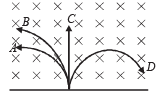Ans. D, B

Solution. According to Fleming's left hand rule, the force on electrons will be towards right (D).

Also, by the same rule we find that the force on proton and a-particle is towards left. Now since the magnetic force will behave as centripetal force, therefore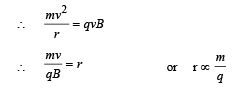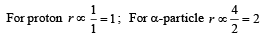∴ radius will be more for α-particle

∴α-particle will take path B.

Q.2. A wire of length L metre, carrying a current i ampere is bent in the form of a circle. The magnitude of its magnetic moment is ........in MKS units.

Ans.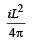Solution.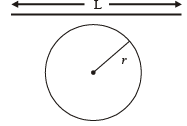Wire of length L is bent in the form of a circle. Then the perimeter of the circle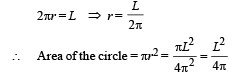Magnetic moment of a loop in which current i flows is given by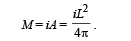Q.3. In a hydrogen atom, the electron moves in an orbit of radius 0.5 Å making 1016 revolutions per second. The magnetic moment associated with the orbital motion of the electron is ......

Ans. 1.25 × 10–23 Am2

Solution.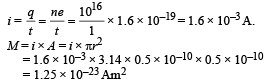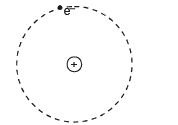Q.4. The wire loop PQRSP formed by joining two semicircular wires of radii R1 and R2 carries a current I as shown. The magnitude of the magnetic induction at the centre C is ....................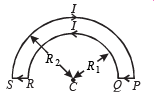Ans.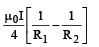Solution. The magnetic field at C due to current in PQ and RS is zero. Magnetic field due to current in semi-circular arc QAR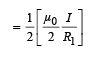directed towards reader perpendicular to the plane of paper.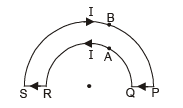Magnetic field due to current in semi-circular arc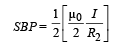directed away from reader perpendicular to the plane of paper.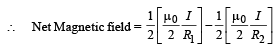(directed towards the reader perpendicular to plane of paper).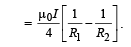Q.5. A wire ABCDEF (with each side of length L) bent as shown in figure and carrying a current I is placed in a uniform magnetic induction B parallel to the positive y-direction.

The force experienced by the wire is ............ in the ............. direction.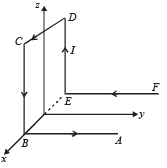Ans. IlB; +Z direction

Solution. We may assume current to be flowing in segment EB in both directions.

Net force on the loop EDCBE will be zero. Also force due to segment FE and BA will be zero. Force due to segment EB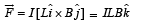Q.6. A metallic block carrying current I is subjected to a uniform magnetic induction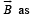shown in Figure .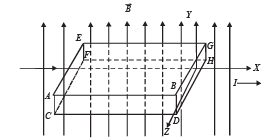The moving charges experience a force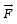given by .... which results in the lowering of the potential of the face .... Assume the speed of the carriers to be v.

Ans. evB; ABCD

Solution.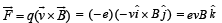NOTE : The direction of flow of electrons is opposite to that of current.

Q.7. No net force acts on a rectangular coil carrying a steady current when suspended freely in a uniform magnetic field.

Ans. T

Solution. A current carrying coil is a magnetic dipole. The net force on a magnetic dipole placed in uniform magnetic field is zero.

Q.8.There is no change in the energy of a  charged particle moving in a magnetic field although a magnetic force is acting on it.

Ans. T

Solution.

NOTE : The magnetic force acts in a direction perpendicular to the direction of velocity and hence it cannot change the speed of the charged particle. Therefore, the kinetic energy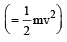does not change.

Q.9. A charged particle enters a region of uniform magnetic field at an angle  of 85° to the magnetic line of force . The path of the particle is a circle.

Ans. F

Solution. The velocity component vwill be responsible in moving the charged particle in a circle.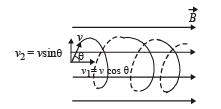The velocity component v1 will be responsible in moving the charged particle in horizontal direction. Therefore the charged particle will travel in a helical path.

Q.10. An electron and a proton are moving with the same kinetic energy along the same direction. When they pass through a uniform magnetic field perpendicular to the direction of their motion, they describe circular paths of the same radius.

Ans. F

Solution. When a charged particle passes through a uniform magnetic field perpendicular to the direction of motion, a force acts on the particle perpendicular to the velocity. This force acts as a centripetal force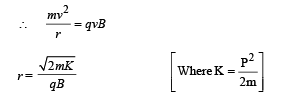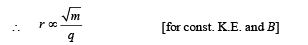Here, q is same for electron and proton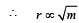Radius of proton will be more.

Offer running on EduRev: Apply code STAYHOME200 to get INR 200 off on our premium plan EduRev Infinity!

42 docs|19 tests

,

,

,

,

,

,

,

,

,

,

,

,

,

,

,

,

,

,

,

,

,

,

,

,

,

,

,

,

,

,

,

,

,

;